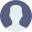# Porkhun : How to implement formula in App Sheet? Step-By-Step Tutorial with Examples

• There are 2 sheet options. The first is “AppSheet” and the second is “Timesheet ”.
• In your case, you need to use the “AppSheet” feature. Timesheet is basically for employee’s time management.
• If you want to perform arithmetic operations then make sure that column type of those columns should be “Number”
• To check the column type and add formula go to App Sheet > Manage Sheets > “click on the “Edit” icon of the desired sheet”.
• To change the field type of any column, go to the “Type” column and click on the dropdown of your desired column and select “Number” from the drop-down.
• To add the formula like in your case DROI calculator, go to “App Formula” column and click on the “Add Formula” option of the sheet’s column in which you want to add the formula.
• On click of “Add Formula”, a pop-up will come, and on pop-up you different tabs like “Math”, “Yes/No”, “Time”, “List”, “Text”, “Other”, select “Math”.
• In the case of “Math” selection, the system will only show the columns which are “Number” type.
• In the “Math” section, you will already get lots of math formulas. By clicking “Insert”, you can use the formula. On click of “Insert”, the formula will be shown in the “Expression builder”
• You can modify the formula but make sure that you enter the correct column name and use “() & []” correctly.
• To save the changes, don’t forget to click on the “Save and Sync” button of the pop-up and after pop-up which comes on the page.

For example, I have created the following formula for ROI calculation.

ROI Calculator:
ARITH(([Amount]-[Unit Price])/[Amount]*100)

Note: Here “Amount” & “Unit Price” are the column names.